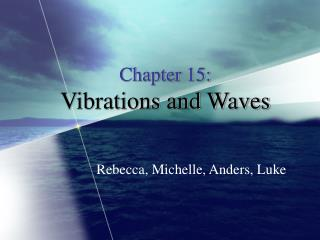DownloadDownload PresentationChapter 15: Vibrations and Waves

# Chapter 15: Vibrations and Waves

Télécharger la présentation## Chapter 15: Vibrations and Waves

- - - - - - - - - - - - - - - - - - - - - - - - - - - E N D - - - - - - - - - - - - - - - - - - - - - - - - - - -
##### Presentation Transcript

1. Chapter 15:Vibrations and Waves Rebecca, Michelle, Anders, Luke

2. Chapter Summary • Vibrations and Oscillations are the length of time needed for each cycle • Period: T=1/f • Frequency: 1/T • Equilibrium Point is where the net force equals zero • The natural frequency of a system resonates with large amplitude • Two types of waves • Transverse: where the vibration of the medium is perpendicular to the motion of the wave produced. • Only in material with rigidity, not in fluid because molecules would slip into one another • Longitudinal: when the vibration of the medium is along the same direction as the motion of the wave. • Can move through most materials because the materials can be compressed and have restoring forces.

3. Summary Continued • Periodic Wave • all pulses have the same size and shape • Wave pattern repeats itself over a distance of 1 wavelength and 1 period • Wavelength: the shortest repetition length for a periodic wave, the distance from crest to crest or trough to trough • Crest: peak point of a wave disturbance • Trough: low point of wave disturbance • Wave Speed: • Multiply the wavelength by the frequency or divide the wavelength by the period v= f or v =  / T

4. Summary Continued • Standing Waves: when there is an interference pattern produced by two waves of equal amplitude and frequency traveling in opposite directions • Node: A position on a standing wave or interference pattern where there is no movement (the amplitude is 0). • Antinode: a position in a standing wave or interference pattern where there is maximal movement (the amplitude is maximum) • Harmonics: frequencies that have a whole-number multiple of the fundamental frequency • The lowest resonant frequency for an oscillating system

5. Problem Areas • Force and acceleration always point towards the equilibrium point. • Waves: • The speed of the pulse doesn’t depend on its size or shape or the manner in which it was created (in a spring). • They key determinants are tension in the object (rope, spring) and the mass of the object. • Pieces or particles in a wave move perpendicular to the motion of the wave not along it. They move up and down within it. White arrows show which way the force and acceleration point.

6. Conceptual Problem Conceptual Question #4A mass is oscillating up and down on a vertical spring. When the mass is below the equilibrium point and moving down, what direction is its acceleration? Is the mass speeding up or slowing down? Answer: a.) The acceleration is in the upward position.b.) The mass is slowing down.

7. What we know: F=1/T T of a pendulum = 2π√(L/G) rope length = 2.5 m weight = 40 kg (useless information) g = 10m/s2 Plug and Chug: T= 2π√(L/G) = 2 π√(2.5m/10m/s2) = 2 π(.5s) = 1 π or just π T= π = 3.14 F = 1/T = 1/π seconds = 0.318 (1/sec) = 3.18 x 10-2 cycles/ second Exercise • A girl with a mass of 40 kg is swinging from a rope with a length of 2.5 m. What is the frequency of her swinging?

8. Pictures found from: • http://commons.wikimedia.org/wiki/Main_Page • http://www.physicsclassroom.com/Class/waves/wavestoc.html • http://id.mind.net/~zona/mstm/physics/waves/partsOfAWave/waveParts.htm